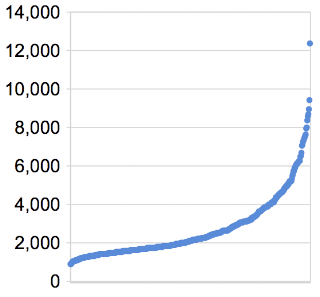# Nicholas Rinard Keene's Little Bit

## Friday, October 2, 2009

### Efficiency Of Genetic AlgorithmLike I did with my first genetic algorithm, I ran the most recent one 500 times overnite. You can see the graph here showing the number of generations it took to reach the optimal state. This algorithm has a bigger tail than the first one, as you can see: although the average was 2693, the median was 2095, and the longest runtime was 12354, six times longer than the median.

I've been thinking about why this algorithm would have that longer tail, and I think it might be because mating between organisms has a lesser impact on the fitness of the offspring. In my first algorithm, a mutation at any point in the string had an equal chance of being a "good" mutation which would increase fitness, because the fitness-evaluation routine considered every character in the string -- that is, every character in the string was always counted either for or against the organism's fitness. Thus, the chance of a positive mutation happening at a certain location, and being selected, is 1 in X, where X is the length of the string. But in this algorithm, the fitness-evaluation routine only considers characters at the left of the string, ignoring characters which occur after the string stops being alphabetical. That means that many mutations which might be positive in a grander sense, such as a 'z' in the very last position, are ignored because they fall outside the comparison zone. So the chance of a positive mutation happening at a certain location, and being selected, is 1 in Y, where Y is the width of the left alphabetized section of the string, and Y is always less than the length of the string, usually quite a bit less.

So I would characterize this algorithm as providing a weaker mechanism of natural selection, thus having a longer tail. That's my best guess, anyway.